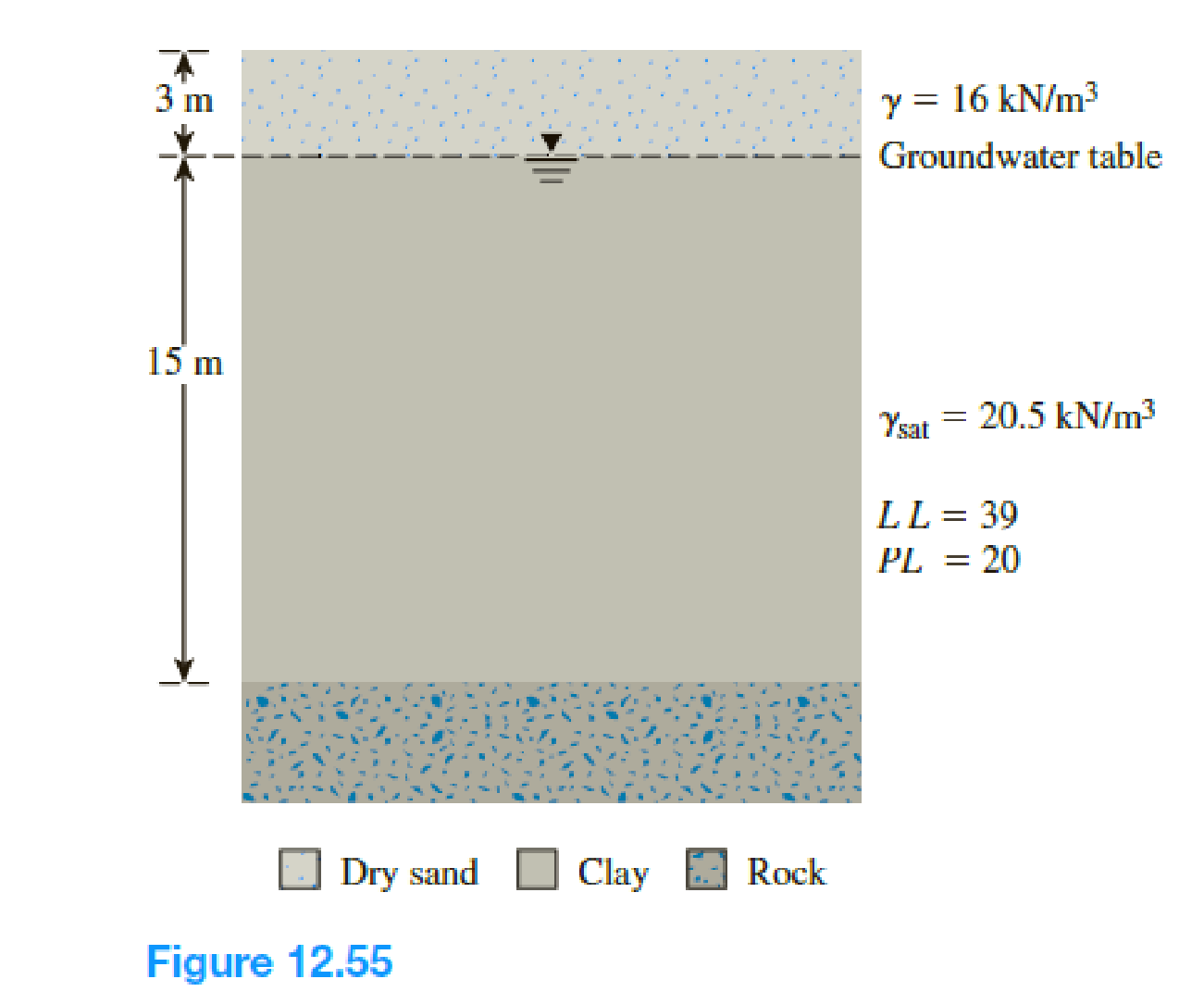Chapter 12, Problem 12.23PPrinciples of Geotechnical Enginee...

9th Edition
Braja M. Das + 1 other
ISBN: 9781305970939

Solutions

Chapter
SectionPrinciples of Geotechnical Enginee...

9th Edition
Braja M. Das + 1 other
ISBN: 9781305970939
Textbook Problem

A 15-m thick normally consolidated clay layer is shown in Figure 12.55. The liquid limit and plastic limit of the soil are 39 and 20, respectively. a. Using Eq. (12.46) given by Skempton (1957), estimate the undrained cohesion at a depth of 11 m below the ground surface as would be obtained by conducting a vane shear test. b. Using Bjerrum’s (1974) correction factor [Eqs. (12.61) and (12.62)], estimate the design value of the undrained shear strength determined in Part a. c. If the clay has the potential of becoming overconsolidated up to OCR = 3.0 due to future ground improvement activities, what would be the new undrained cohesion? Use Eq. (12.47) given by Ladd et al. (1977).(a)

To determine

Find the undrained cohesion at a depth of 11m below the ground surface.

Explanation

Given information:

The liquid limit (LL) of the soil is 39.

The plastic limit (PL) of the soil is 20.

The saturated (γsat) unit weight of clay is 20.5kN/m2

The depth (H1) of the sand above the water table is 3m.

The unit weight (γ) of dry sand is 16kN/m2.

Calculation:

Find the plasticity index (PI) using the relation.

PI=LLPL (1)

Substitute 39 for (LL) and 20 for (PL) in Equation (1).

PI=3920=19

Show the empirical relationship between undrained cohesion (cu) and effective overburden pressure (σo) as follows:

cu(VST)σo=0.11+0.0037(PI) (2)

Here, cu(VST) is undrained shear strength from vane shear test and (σo) is overburden pressure.

Substitute 19 for PI in Equation (2).

cu(VST)σo=0.11+0.0037(19)cu(VST)σo=0.1803 (3)

Calculate the overburden pressure (σo) using the relation.

σo=H1(γ)+H2(γsatγw) (4)

Here, H2 is depth of the clay

(b)

To determine

Find the design value of undrained shear strength by using Bjerrum’s correction factor.

(c)

To determine

Find the new undrained cohesion.

Still sussing out bartleby?

Check out a sample textbook solution.

See a sample solution

The Solution to Your Study Problems

Bartleby provides explanations to thousands of textbook problems written by our experts, many with advanced degrees!

Get Started

What is the main difference between learning in high school and college?

Engineering Fundamentals: An Introduction to Engineering (MindTap Course List)

What is an extranet and how is one used?

Principles of Information Systems (MindTap Course List)

What does SAE stand for?

Precision Machining Technology (MindTap Course List)

How do you translate business rules into data model components?

Database Systems: Design, Implementation, & Management

Solid Waste Engineering

Name five different types of welding career opportunities.

Welding: Principles and Applications (MindTap Course List)